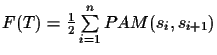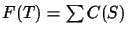Next: Finding a circular order Up: Methods Previous: Traveling Salesman

## Scoring an Evolutionary Tree

Since traversing a tree T(S) in circular order counts each edge in the tree exactly twice, we can derive a function for determining the score of an evolutionary tree using this order. First, we reorder the sequences in S according to the circular order C(S). If we now add the PAM distances of the pairwise alignments in this order and divide this sum by two, we score each edge of the tree once. So the function for scoring a tree T(S) is simple:where sn+1 = s1, n is the number of sequences, and PAM is the PAM distance of the optimal pairwise alignment (see Definition 1.3). We abbreviate this function withChantal Korostensky
1999-07-14